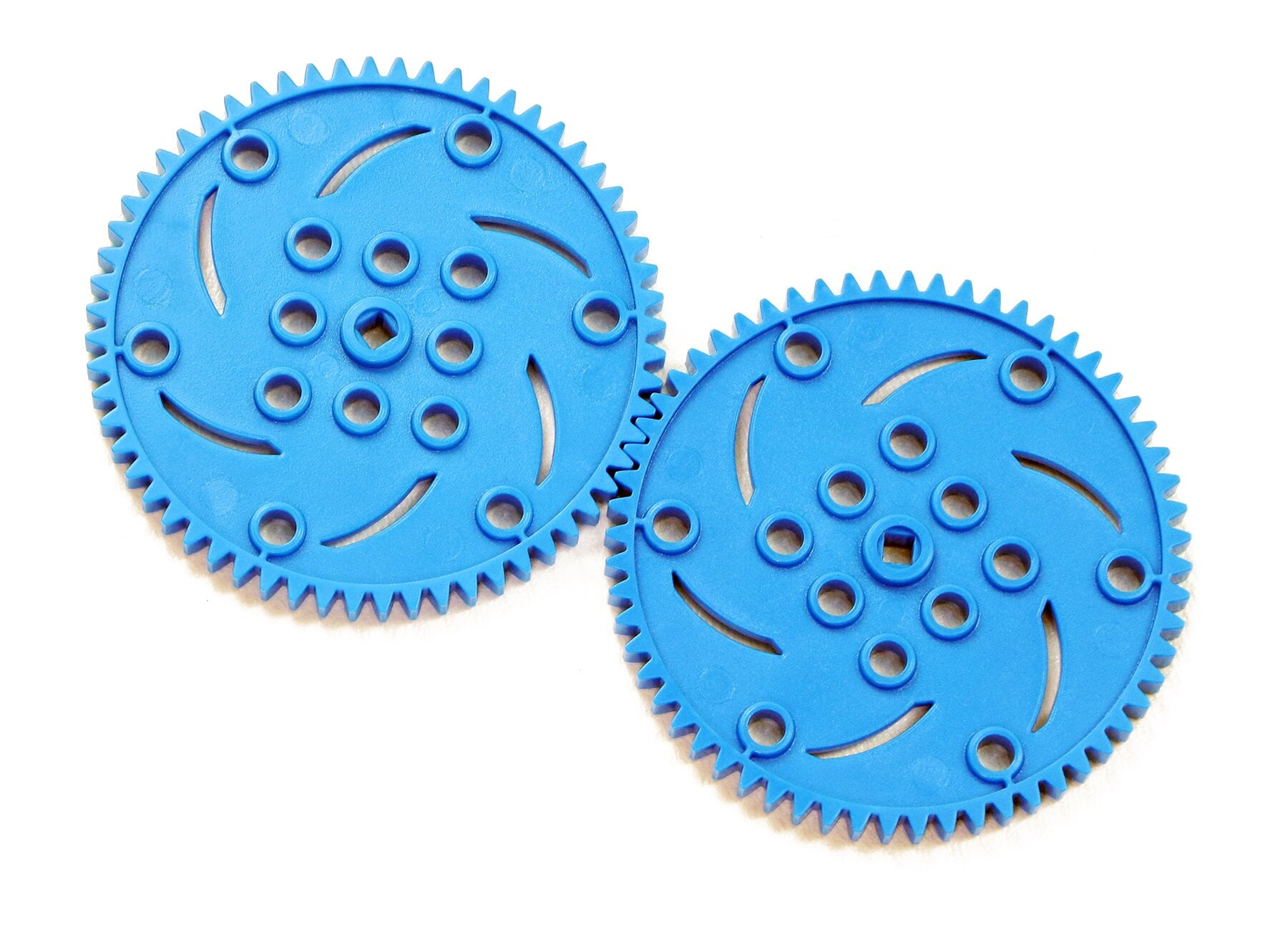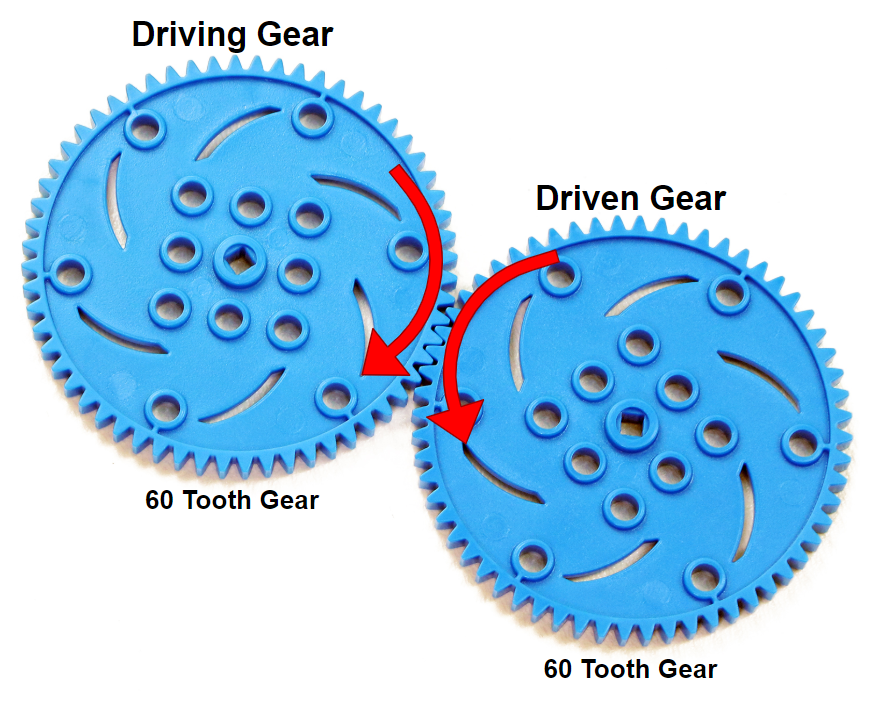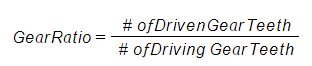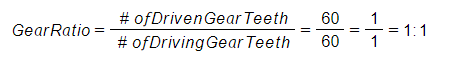STEMLabs IQ
Lab 12 - M.A.D. Box

Teacher Portal

# What are Gears?Teacher Toolbox - The Purpose of this Section

The Play section of this STEM Lab will introduce students to gears and their purpose within a build. This first page will introduce terms like: meshed gears, driving gears, driven gears, gear ratios, mechanical advantage, and power transfer. The next page will review the build of the M.A.D. Box, its gear ratios, and the mechanical advantages of either torque or speed that are created by those ratios. The next page culminates in the calculation of the gear ratio for the entire M.A.D. Box build.
It may be a good idea to read through the first page as a class and then have student groups work through the second page. Every student should be reading along.

If working in groups of three, students can be assigned to one of three roles:

1) The Build Expert: This student finds the build's step in the Build Instructions and points out where in the completed build those gears are located.

2) The Calculator: This student carries out the calculations of the gear ratios.

3) The Recorder: This student checks the math of the Calculator, interprets what the gear ratio means, and ensures that the team is recording all of their work within their engineering notebook.

If groups of students have more than three students, then multiple students could be responsible for the same role. If groups have fewer than three, the same student can assume multiple roles.

Click here for the Collaboration rubric, or click here for the group-based engineering notebook rubric. There is also an Individual engineering notebook rubric, click here. Any rubrics that will be used for scoring should be shared with and explained to students before work begins.### Gears

Gears look like disks with teeth around their edges. It is important to notice that their teeth are equally spaced because gears work by having their teeth meshed together, as shown in the image above. When one gear turns, it turns the next one because their teeth are positioned between each other, which is known as being meshed.

Gears are typically mounted, or connected to other parts, by a shaft or base. So gears are used to transmit rotary motion, or power, from one shaft to another. The shaft is usually positioned at the gear's center. In the image above of the VEX IQ Gears, the center hole to pass a shaft through is the square one because the IQ Shafts are square.

One of the main ways to define a gear is by the number of teeth that it has.Teacher Tips

It may help to direct students to the VEX IQ Parts poster and have them notice that the gears included in the Super Kit are named using their numbers of teeth (60, 36, and 12 Tooth Gears). If students are confused by the mounting of gears on shafts, they can be directed to look at the M.A.D. Box build and notice that all over the gears within the build were built onto shafts at their centers.

### Meshed Gears

When two gears are meshed together, one gear turns the next. The gear that is doing the turning first is called the driving gear. The driving gear can be thought of as a type of input. The gear that is being turned by the first gear is called the driven gear. The driven gear is therefore the output.

Watch the animation below to see meshed gears in action.

Video file

You should have noticed that the driving gear and driven gear turn in opposite directions. They have to spin in opposite directions because their teeth are meshed and they rotate at their centers.

### Gear RatiosA gear ratio is a comparison of the input (driving gear) to output (driven gear) and is calculated by considering each meshed gear's number of teeth.

In the example above, the driving gear (input) and the driven gear (output) both have 60 teeth.

Here is the formula for calculating a gear ratio:Let's use the example of the two 60 Tooth Gears above because it's a simple ratio to calculate.The gear ratio of these two meshed gears is 1:1 which means each time the driving gear (input) turns one full rotation, the driven gear (output) also turns one full rotation.Teacher Tips

Ensure that students recognize what ratios are from this or another class. Simply stated, a ratio is a statement of how two numbers compare.

Also, ensure that students understand how to reduce fractions by dividing the numerator and denominator by the same amount.

Whenever two or more gears are meshed, a mechanical advantage is created within that build.

Mechanical advantage is defined as the change of input force within a machine. The change can be measured by comparing the input and output.

In the example above, the input and output have a 1:1 ratio so it might seem like there is no mechanical advantage but there actually is. The mechanical advantage when two gears are the same size is called power transfer because the driven gear and its shaft turn just as much as the driving gear and its shaft. So the driving gear (input) transferred all of its power to the driven gear (output).

In the next activity, you will review your M.A.D. Box build and will calculate and test the mechanical advantages of speed and torque.Extend Your Learning - Gears in Daily Life

Many mechanical devices use gears. In the digital age, there might seemingly be fewer but students should be able to identify at least five devices that use gears. They can investigate tools prior to electricity as well. Students should then explain how each uses gears, and what the gear system's function(s) is.

Here are some examples:

• Many bicycles use gears to allow the rider to shift gears to pedal faster or with more force.

• Hand-mixers in the kitchen, prior to electricity, used gears so that the user could turn a handled crank in one direction (e.g., up and down) and have the beaters of the mixer spin in another (e.g., back and forth) in the bowl of ingredients.

• Watermills also used gears to change the direction of the force through power transfer. The water would turn the turbine (water wheel) which would turn gears, transferring power into the factory where it was used to mill, roll, or hammer products.